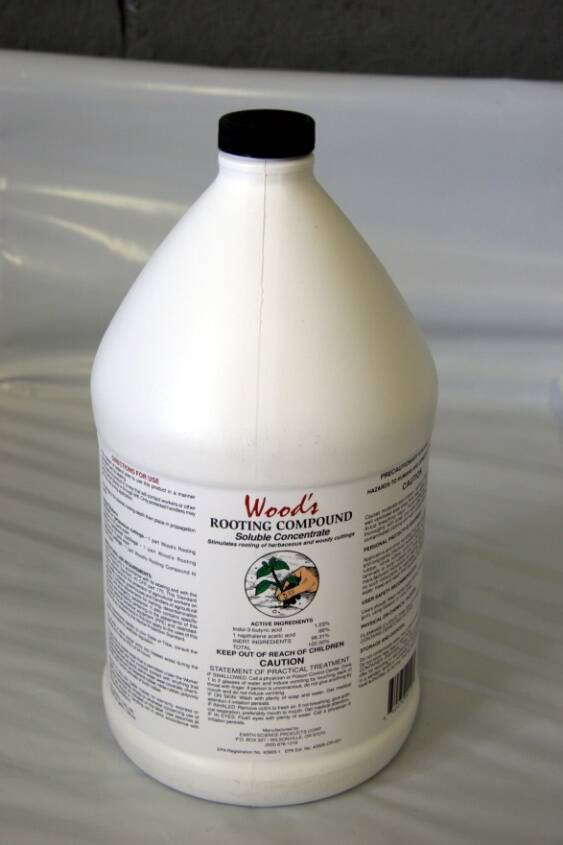A part per million (ppm) is a way to measure concentrations of a solvent in a solution.

It is a typical way propagator's calculate hormone concentrations used to treat cuttings to improve rooting or measure out fertilizers.

To make a solution, a quantity of solute is added to a known volume of solvent. As an example, imagine adding 10 mg of the rooting hormone IBA (the solute) to 1liter of ethanol (the solvent).

This will make a 10 ppm solution. There are 10 parts of IBA per 1 million parts of ethanol.

One part per million is one mg of solute per liter of solvent.

A 5000 ppm solution of IBA = 5000 mg of IBA per liter of solvent.

(a typical solvent used for treating cuttings is 50% ethanol).

Another way to calculate a hormone concentration is by percentage.

A 2% solution = 20,000 ppm

20,000 ppm = 20,000 mg per liter

Commercial hormone formulations are sold as concentrated stock solutions usually with alcohol as the solvent.

Typical solutions include:

Dip 'N Grow = 1% IBA + 2% NAA

Wood's rooting compound = 1.03% IBA + 0.56% NAATypical solution concentrations used for quick dip treatments are dilutions from commercial concentrated preparations made in 50% alcohol or water.

Use 500 to 1,250 ppm for easy-to-root herbaceous cuttings.

Use 500 to 3,000 ppm for easy-to-root woody cuttings.

Use up to 10,000 ppm for difficult-to-root woody cuttings.

The basic formula for calculating a final solution from a commercial concentrate is the following dilution:

PPM of concentrate x Volume to be removed from concentrate = Desired PPM of new solution x Volume needed for the new solution.

Make a 1 liter solution containing 250 ppm IBA from an auxin concentrate that contains 1% IBA.

The basic formula for calculating a final solution from a commercial concentrate is the following dilution:

PPM of concentrate x Volume to be removed from concentrate = Desired PPM of new solution x Volume needed for the new solution.

Make a 1 liter solution containing 250 ppm IBA from an auxin concentrate that contains 1% IBA.

1. Solve for volume
10,000 ppm X Vol (ml) = 250 ppm X 1000 ml
2. Divide both sides by 10,000 ppm
Vol (ml) = 250 ppm X 1000 ml / 10,000 ppm
3. Multiply 250 ppm by 1000 ml
Vol (ml) = 250,000 ppm ml / 10,000 ppm
4. Divide by 10,000 ppm
Vol (ml) = 250,000 ppm ml / 10,000 ppm
5. Add 25 ml of concentrate to 1 liter
Vol (ml) = 25 ml